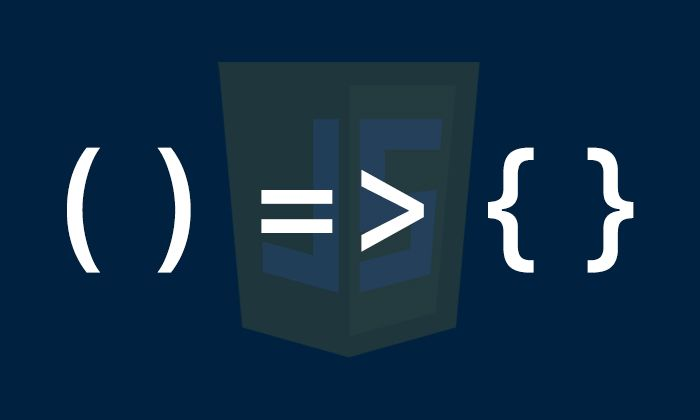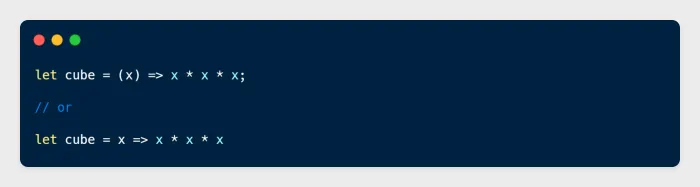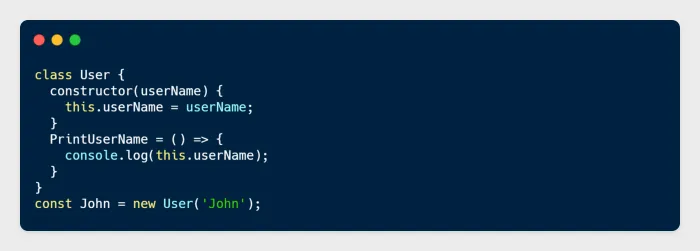# How and Where We use Arrow Function and Regular Function?In this article, you’ll learn all about JavaScript arrow functions and regular functions and what is the major difference between them and when we should use one over the other. You’ll see lots of examples that illustrate how they work.

## 1. Syntax Difference

Functions are like recipes where you store useful instructions to accomplish something you need to happen in your program, like performing an action or returning a value. By calling your function, you execute the steps included in your recipe. You can do so every time you call that function without needing to rewrite the recipe again and again.

### a) Regular Function

Here’s the standard way of declaration of a regular function and calling that function in Javascript.

```let HelloWorld = function () {
return 'Hello World';
}```

### b) Arrow Function

JavaScript arrow functions are also called Fat arrow functions. These were introduced in ES6. Their concise syntax and handling of the `this` keyword has made JavaScript arrow functions an ES6 favourite among developers.

`() => 'Hello';`
`_ => 'Hello';`iii) Multiple Parameter

`let add = (x, y) => x + y;`
```let add = (x, y) => {
let z = 10;
return x + y + z;
}```

## 2. Syntactically Anonymous

It is important to note that arrow functions are anonymous, which means that they are not named. This will create some issues listed below.

### a) Harder to debug

When you get an error, you will not be able to trace the name of the function or the exact line number where it occurred.

### b) No self-Referencing

If your function needs to have a self-reference at any point (e.g. recursion, an event handler that needs to unbind), it will not work.

## 3. Use of “`new”` keyword

### a) Regular Function

Regular functions are constructible, so they can be called using the `new` keyword.

### b) Arrow Function

On the other hand, Arrow functions are not constructible so the arrow function will never use as a constructor. That’s why arrow functions are never used with `new` keyword.

## 4. Use of “this” keyword

### a) Regular Function

We can use `this` keyword in regular function and we can access all the variables and functions using `this` .

### b) Arrow Function

But on the other hand, we can not use `this` keyword directly into arrow function because it does not contain its own `this` . So when we use `this` keyword in the arrow function, it will return an undefined value.

## 5. Instantiating a function

We create an empty object inside a function and then we add some properties and functions into that object and then we return that object.

## 7. Returning Values

### a) Regular Function

`return` key is used to return value or statement in the regular functions as given below.

### b) Arrow Function

We can return values from the arrow function the same way as from a regular function, but with one useful exception.

## 8. Methods

### a) Regular Function

We can check from the following example in which `PrintUserName()`is defined in class `User`

### b) Arrow Function

Now, in contrast with regular functions, the method defined using an arrow binds `this` lexically to the class instance.

####